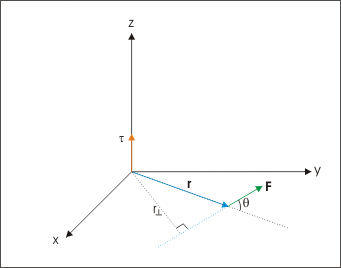$$\require{cancel}$$

# 10.9: Introduction

•• Boundless
• Boundless
$$\newcommand{\vecs}{\overset { \rightharpoonup} {\mathbf{#1}} }$$ $$\newcommand{\vecd}{\overset{-\!-\!\rightharpoonup}{\vphantom{a}\smash {#1}}}$$$$\newcommand{\id}{\mathrm{id}}$$ $$\newcommand{\Span}{\mathrm{span}}$$ $$\newcommand{\kernel}{\mathrm{null}\,}$$ $$\newcommand{\range}{\mathrm{range}\,}$$ $$\newcommand{\RealPart}{\mathrm{Re}}$$ $$\newcommand{\ImaginaryPart}{\mathrm{Im}}$$ $$\newcommand{\Argument}{\mathrm{Arg}}$$ $$\newcommand{\norm}{\| #1 \|}$$ $$\newcommand{\inner}{\langle #1, #2 \rangle}$$ $$\newcommand{\Span}{\mathrm{span}}$$ $$\newcommand{\id}{\mathrm{id}}$$ $$\newcommand{\Span}{\mathrm{span}}$$ $$\newcommand{\kernel}{\mathrm{null}\,}$$ $$\newcommand{\range}{\mathrm{range}\,}$$ $$\newcommand{\RealPart}{\mathrm{Re}}$$ $$\newcommand{\ImaginaryPart}{\mathrm{Im}}$$ $$\newcommand{\Argument}{\mathrm{Arg}}$$ $$\newcommand{\norm}{\| #1 \|}$$ $$\newcommand{\inner}{\langle #1, #2 \rangle}$$ $$\newcommand{\Span}{\mathrm{span}}$$

learning objectives

• Describe the effect of the torque on an object

Torque about a point is a concept that denotes the tendency of force to turn or rotate an object in motion. This tendency is measured in general about a point, and is termed as moment of force. The torque in angular motion corresponds to force in translation. It is the “cause” whose effect is either angular acceleration or angular deceleration of a particle in general motion. Quantitatively, it is defined as a vector given by:

Torque: A brief introduction to torque for students studying rotational motion in algebra-based physics courses such as AP Physics 1 and Honors Physics.

$mathrm{T=r \times F}$

Rotation is a special case of angular motion. In the case of rotation, torque is defined with respect to an axis such that vector “r” is constrained as perpendicular to the axis of rotation. In other words, the plane of motion is perpendicular to the axis of rotation. Clearly, the torque in rotation corresponds to force in translation.

Torque is the cross product of force cross length of the moment arm; it is involved whenever there is a rotating object. Torque can also be expressed in terms of the angular acceleration of the object.

The determination of torque’s direction is relatively easier than that of angular velocity. The reason for this is simple: the torque itself is equal to vector product of two vectors, unlike angular velocity which is one of the two operands of the vector product. Clearly, if we know the directions of two operands here, the direction of torque can easily be interpreted.

Since torque depends on both the force and the distance from the axis of rotation, the SI units of torque are newton-meters.Torque: Torque in terms of moment arm.

## Key Points

• Torque is found by multiplying the applied force by the distance to the axis of rotation, called the moment arm.
• Torque is to rotation as force is to motion.
• The unit of torque is the newton-meter.

## Key Terms

• vector: A directed quantity, one with both magnitude and direction; the between two points.
• angular velocity: A vector quantity describing an object in circular motion; its magnitude is equal to the speed of the particle and the direction is perpendicular to the plane of its circular motion.
• angular motion: The motion of a body about a fixed point or fixed axis (as of a planet or pendulum). It is equal to the angle passed over at the point or axis by a line drawn to the body.